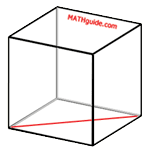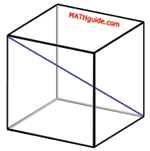Diagonal of a Cube
View the Lesson | MATHguide homepage Updated January 7th, 2022

 Given: The length of an edge of a cube is 4 units. Find: a) The length of the diagonal of its face.df = units b) The length of its internal diagonal.di = units